# FriendlyCore: A novel differentially private aggregation framework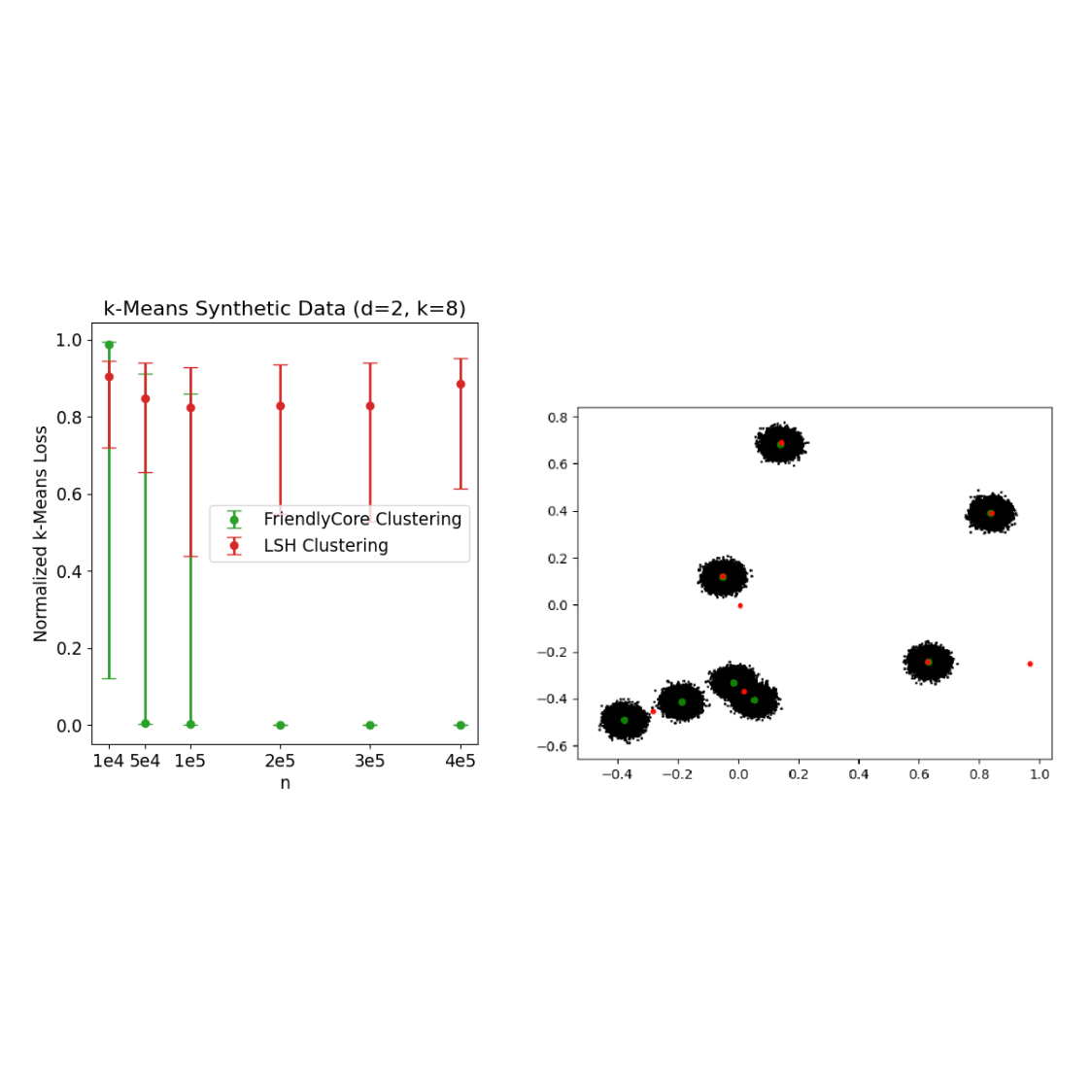Differential privacy (DP) machine learning algorithms protect user data by limiting the effect of each data point on an aggregated output with a mathematical guarantee. Intuitively the guarantee implies that changing a single user’s contribution should not significantly change the output distribution of the DP algorithm.

However, DP algorithms tend to be less accurate than their non-private counterparts because satisfying DP is a worst-case requirement: one has to add noise to “hide” changes in any potential input point, including "unlikely points’’ that have a significant impact on the aggregation. For example, suppose we want to privately estimate the average of a dataset, and we know that a sphere of diameter, Λ, contains all possible data points. The sensitivity of the average to a single point is bounded by Λ, and therefore it suffices to add noise proportional to Λ to each coordinate of the average to ensure DP.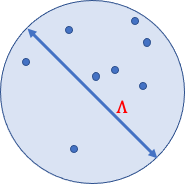A sphere of diameter Λ containing all possible data points.

Now assume that all the data points are "friendly," meaning they are close together, and each affects the average by at most 𝑟, which is much smaller than Λ. Still, the traditional way for ensuring DP requires adding noise proportional to Λ to account for a neighboring dataset that contains one additional "unfriendly" point that is unlikely to be sampled.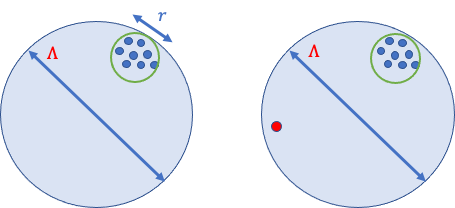Two adjacent datasets that differ in a single outlier. A DP algorithm would have to add noise proportional to Λ to each coordinate to hide this outlier.

In “FriendlyCore: Practical Differentially Private Aggregation”, presented at ICML 2022, we introduce a general framework for computing differentially private aggregations. The FriendlyCore framework pre-processes data, extracting a “friendly” subset (the core) and consequently reducing the private aggregation error seen with traditional DP algorithms. The private aggregation step adds less noise since we do not need to account for unfriendly points that negatively impact the aggregation.

In the averaging example, we first apply FriendlyCore to remove outliers, and in the aggregation step, we add noise proportional to 𝑟 (not Λ). The challenge is to make our overall algorithm (outlier removal + aggregation) differentially private. This constrains our outlier removal scheme and stabilizes the algorithm so that two adjacent inputs that differ by a single point (outlier or not) should produce any (friendly) output with similar probabilities.

## FriendlyCore Framework

We begin by formalizing when a dataset is considered friendly, which depends on the type of aggregation needed and should capture datasets for which the sensitivity of the aggregate is small. For example, if the aggregate is averaging, the term friendly should capture datasets with a small diameter.

To abstract away the particular application, we define friendliness using a predicate 𝑓 that is positive on points 𝑥 and 𝑦 if they are “close” to each other. For example,in the averaging application 𝑥 and 𝑦 are close if the distance between them is less than 𝑟. We say that a dataset is friendly (for this predicate) if every pair of points 𝑥 and 𝑦 are both close to a third point 𝑧 (not necessarily in the data).

Once we have fixed 𝑓 and defined when a dataset is friendly, two tasks remain. First, we construct the FriendlyCore algorithm that extracts a large friendly subset (the core) of the input stably. FriendlyCore is a filter satisfying two requirements: (1) It has to remove outliers to keep only elements that are close to many others in the core, and (2) for neighboring datasets that differ by a single element, 𝑦, the filter outputs each element except 𝑦 with almost the same probability. Furthermore, the union of the cores extracted from these neighboring datasets is friendly.

The idea underlying FriendlyCore is simple: The probability that we add a point, 𝑥, to the core is a monotonic and stable function of the number of elements close to 𝑥. In particular, if 𝑥 is close to all other points, it’s not considered an outlier and can be kept in the core with probability 1.

Second, we develop the Friendly DP algorithm that satisfies a weaker notion of privacy by adding less noise to the aggregate. This means that the outcomes of the aggregation are guaranteed to be similar only for neighboring datasets 𝐶 and 𝐶' such that the union of 𝐶 and 𝐶' is friendly.

Our main theorem states that if we apply a friendly DP aggregation algorithm to the core produced by a filter with the requirements listed above, then this composition is differentially private in the regular sense.

## Clustering and other applications

Other applications of our aggregation method are clustering and learning the covariance matrix of a Gaussian distribution. Consider the use of FriendlyCore to develop a differentially private k-means clustering algorithm. Given a database of points, we partition it into random equal-size smaller subsets and run a good non-private k-means clustering algorithm on each small set. If the original dataset contains k large clusters then each smaller subset will contain a significant fraction of each of these k clusters. It follows that the tuples (ordered sets) of k-centers we get from the non-private algorithm for each small subset are similar. This dataset of tuples is expected to have a large friendly core (for an appropriate definition of closeness).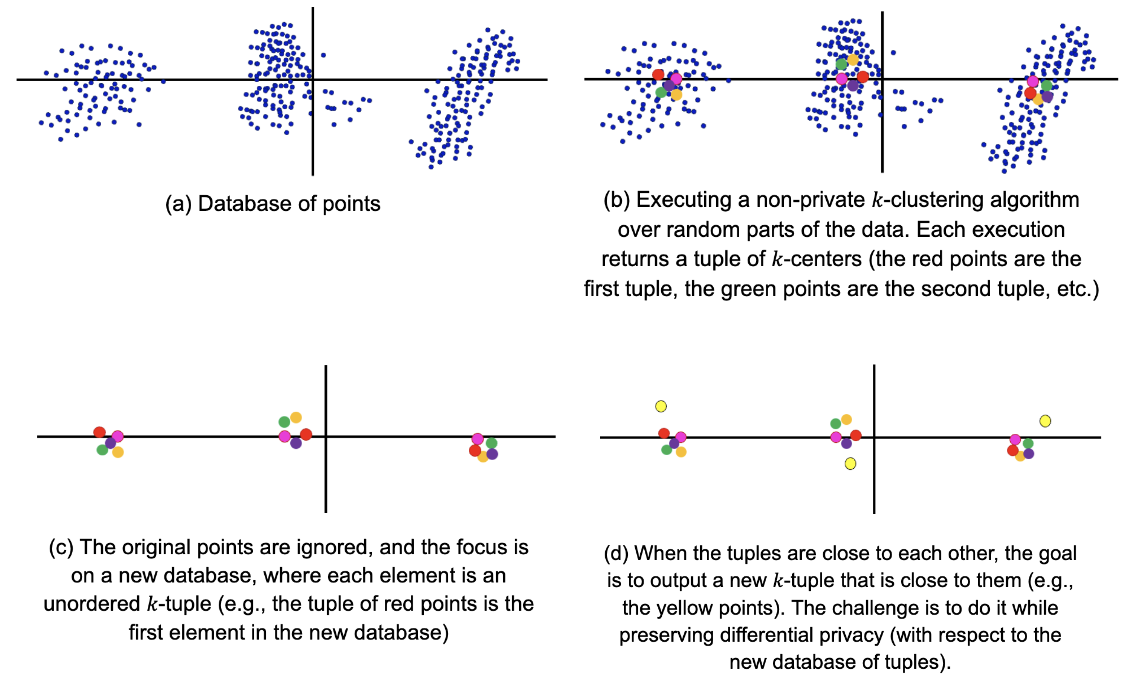We use our framework to aggregate the resulting tuples of k-centers (k-tuples). We define two such k-tuples to be close if there is a matching between them such that a center is substantially closer to its mate than to any other center.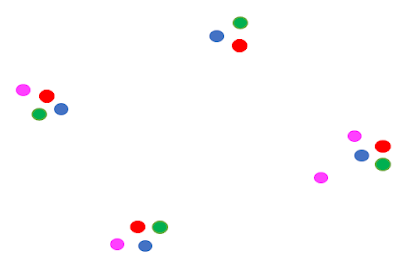In this picture, any pair of the red, blue, and green tuples are close to each other, but none of them is close to the pink tuple. So the pink tuple is removed by our filter and is not in the core.

We then extract the core by our generic sampling scheme and aggregate it using the following steps:

1. Pick a random k-tuple 𝑇 from the core.
2. Partition the data by putting each point in a bucket according to its closest center in 𝑇.
3. Privately average the points in each bucket to get our final k-centers.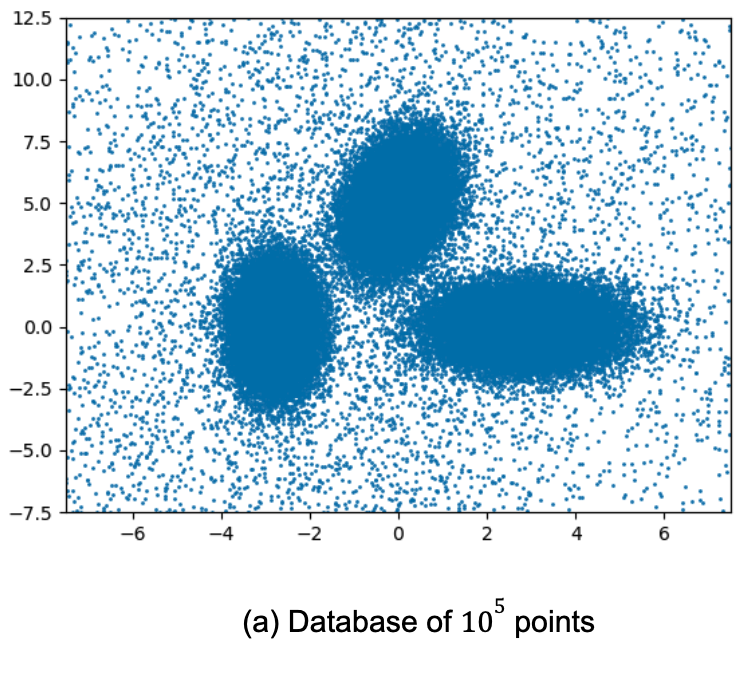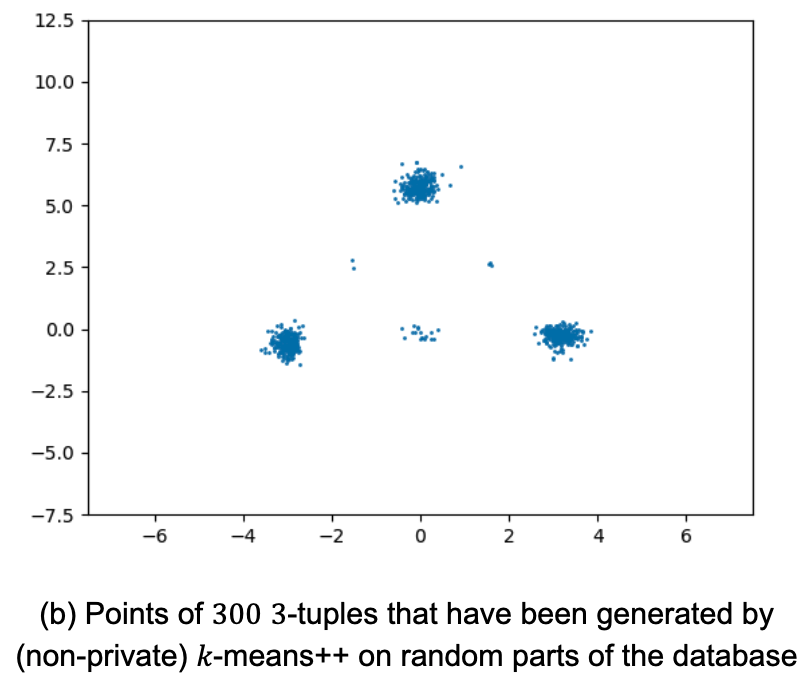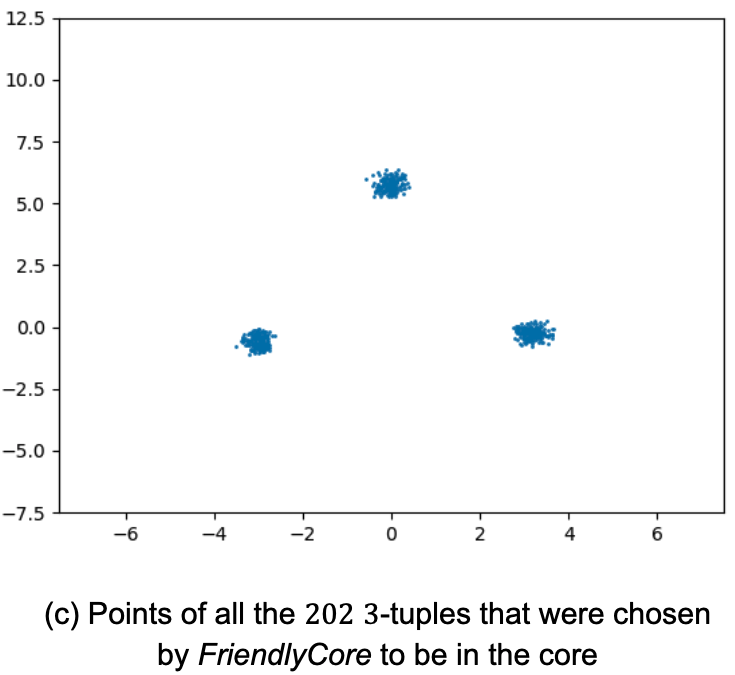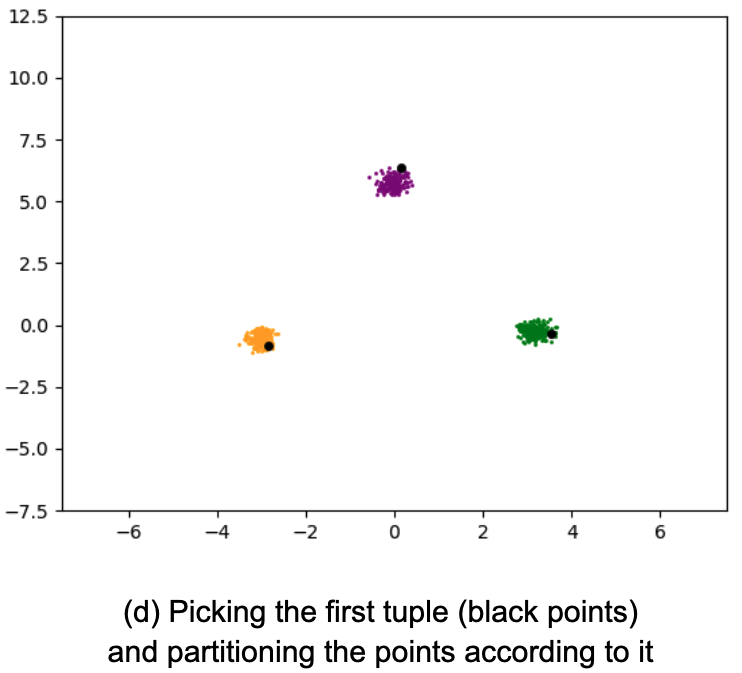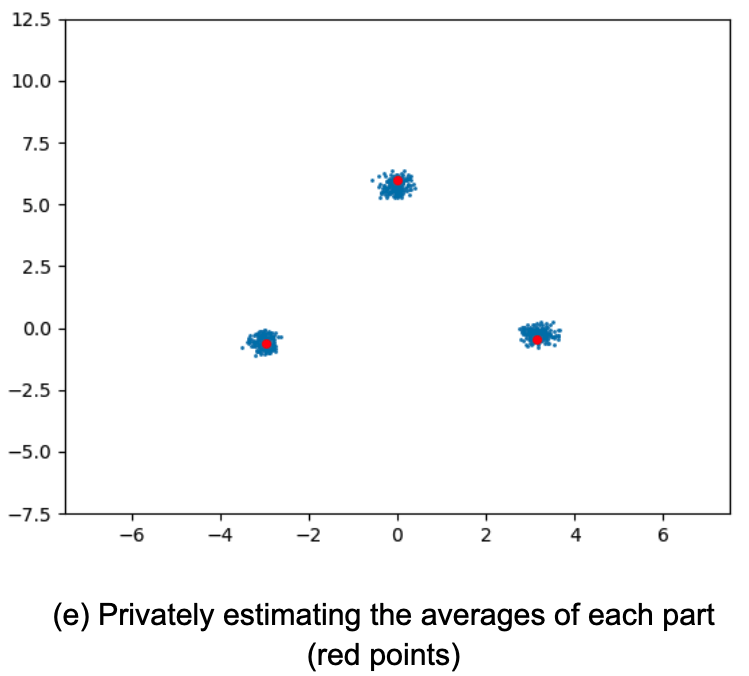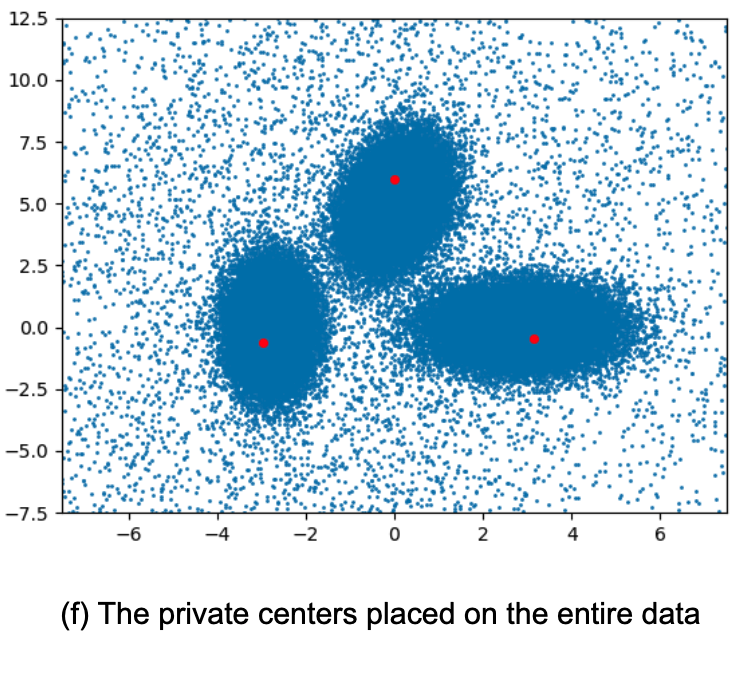## Empirical results

Below are the empirical results of our algorithms based on FriendlyCore. We implemented them in the zero-Concentrated Differential Privacy (zCDP) model, which gives improved accuracy in our setting (with similar privacy guarantees as the more well-known (𝜖, 𝛿)-DP).

### Averaging

We tested the mean estimation of 800 samples from a spherical Gaussian with an unknown mean. We compared it to the algorithm CoinPress. In contrast to FriendlyCore, CoinPress requires an upper bound 𝑅 on the norm of the mean. The figures below show the effect on accuracy when increasing 𝑅 or the dimension 𝑑. Our averaging algorithm performs better on large values of these parameters since it is independent of 𝑅 and 𝑑.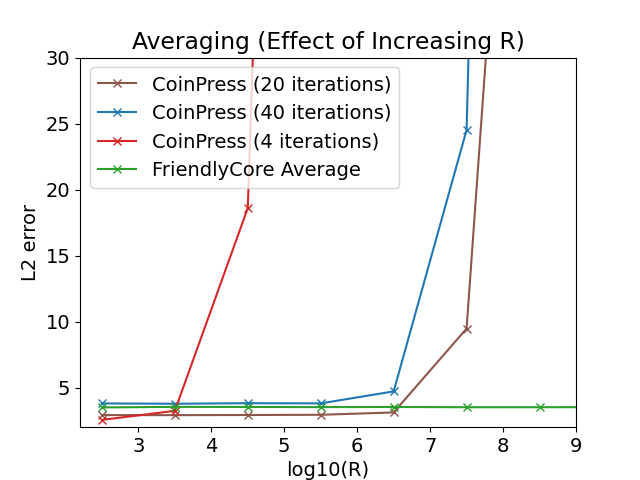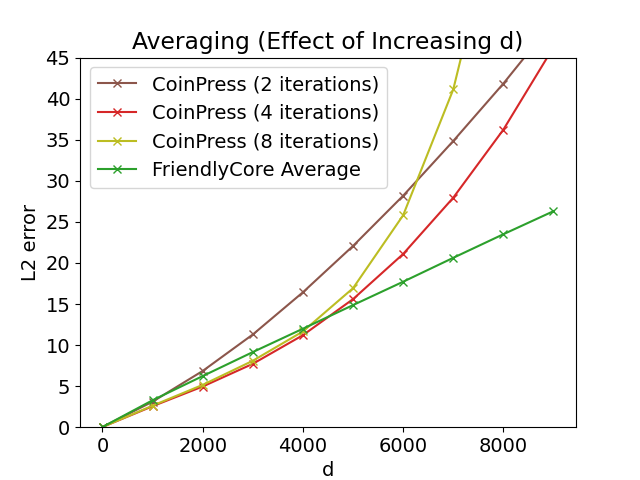Left: Averaging in 𝑑= 1000, varying 𝑅. Right: Averaging with 𝑅= √𝑑, varying 𝑑.

### Clustering

We tested the performance of our private clustering algorithm for k-means. We compared it to the Chang and Kamath algorithm that is based on recursive locality-sensitive hashing (LSH-clustering). For each experiment, we performed 30 repetitions and present the medians along with the 0.1 and 0.9 quantiles. In each repetition, we normalize the losses by the loss of k-means++ (where a smaller number is better).

The left figure below compares the k-means results on a uniform mixture of eight separated Gaussians in two dimensions. For small values of 𝑛 (the number of samples from the mixture), FriendlyCore often fails and yields inaccurate results. Yet, increasing 𝑛 increases the success probability of our algorithm (because the generated tuples become closer to each other) and yields very accurate results, while LSH-clustering lags behind.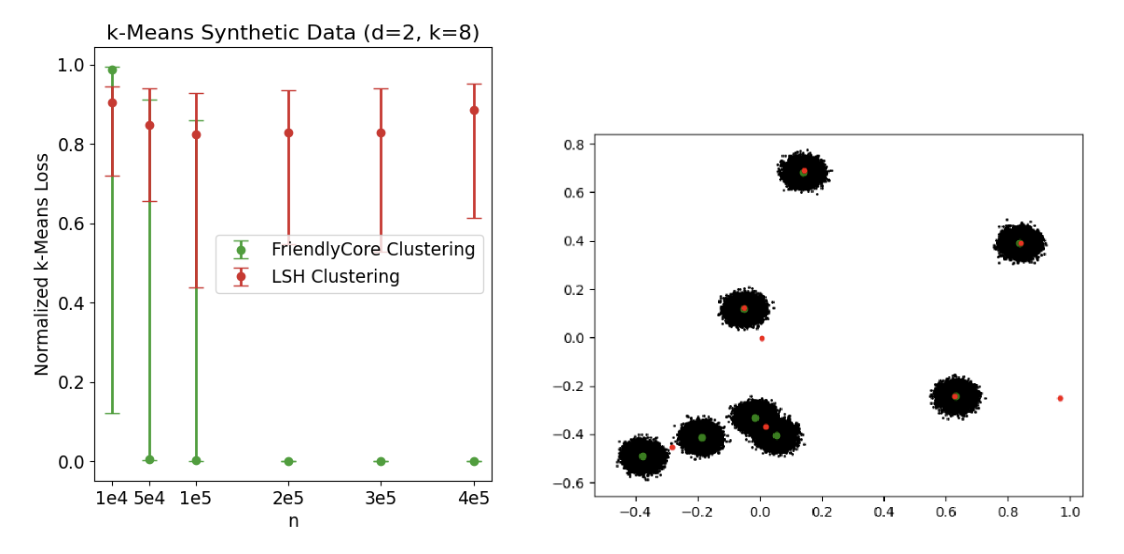Left: k-means results in 𝑑= 2 and k= 8, for varying 𝑛(number of samples). Right: A graphical illustration of the centers in one of the iterations for 𝑛= 2 X 105. Green points are the centers of our algorithm and the red points are the centers of LSH-clustering.

FriendlyCore also performs well on large datasets, even without clear separation into clusters. We used the Fonollosa and Huerta gas sensors dataset that contains 8M rows, consisting of a 16-dimensional point defined by 16 sensors' measurements at a given point in time. We compared the clustering algorithms for varying k. FriendlyCore performs well except for k= 5 where it fails due to the instability of the non-private algorithm used by our method (there are two different solutions for k= 5 with similar cost that makes our approach fail since we do not get one set of tuples that are close to each other).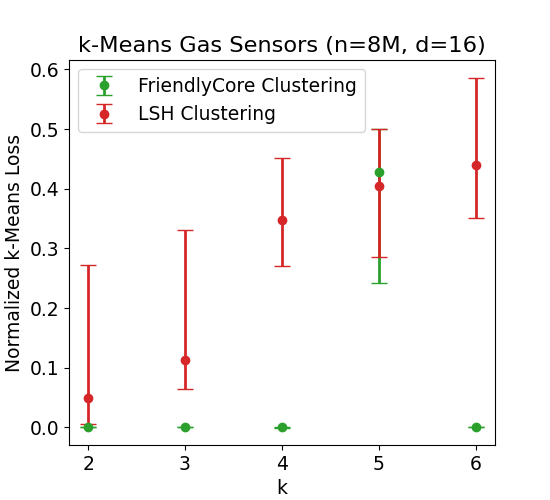k-means results on gas sensors' measurements over time, varying k.

## Conclusion

FriendlyCore is a general framework for filtering metric data before privately aggregating it. The filtered data is stable and makes the aggregation less sensitive, enabling us to increase its accuracy with DP. Our algorithms outperform private algorithms tailored for averaging and clustering, and we believe this technique can be useful for additional aggregation tasks. Initial results show that it can effectively reduce utility loss when we deploy DP aggregations. To learn more, and see how we apply it for estimating the covariance matrix of a Gaussian distribution, see our paper.

## Acknowledgements

This work was led by Eliad Tsfadia in collaboration with Edith Cohen, Haim Kaplan, Yishay Mansour, Uri Stemmer, Avinatan Hassidim and Yossi Matias.